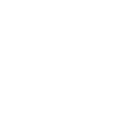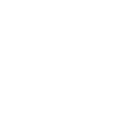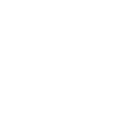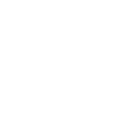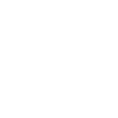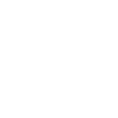# Chapter 11 Programming

## 11.1 Part dimensions

Programming with flextable can be made easier by using a few functions:

• `nrow_part()` returns the number of lines in a part of flextable.
• `ncol_keys()` returns the number of columns displayed.

For example, if you want to add an aggregation line below a sample and to format it differently from the sample rows :

``````library(flextable)
library(dplyr)
library(tibble)

dat_summary <- summarize(airquality, across(Ozone:Temp, ~ mean(.x, na.rm = TRUE))) %>%
add_column(label = "summary", .before = TRUE)
dat_summary``````
``````#     label    Ozone  Solar.R     Wind     Temp
# 1 summary 42.12931 185.9315 9.957516 77.88235``````
``````dat_sample <- head(airquality) %>%
add_column(label = "sample", .before = TRUE)
dat_sample``````
``````#    label Ozone Solar.R Wind Temp Month Day
# 1 sample    41     190  7.4   67     5   1
# 2 sample    36     118  8.0   72     5   2
# 3 sample    12     149 12.6   74     5   3
# 4 sample    18     313 11.5   62     5   4
# 5 sample    NA      NA 14.3   56     5   5
# 6 sample    28      NA 14.9   66     5   6``````
``````dat <- bind_rows(dat_sample, dat_summary)

ft <- flextable(dat, col_keys = colnames(airquality)) %>%
colformat_double(digits = 2, j = colnames(airquality)) %>%
theme_booktabs() %>%
bold(i = nrow_part(., part = "body")) %>%
footnote(i = nrow_part(., part = "body"), j = 1:4,
value = as_paragraph("Calculated mean"),
ref_symbols = c("(1)")) %>%
set_caption("a *weird* table summary")

ft``````
Table 11.1: a weird table summary

Ozone

Solar.R

Wind

Temp

Month

Day

41.00

190.00

7.40

67.00

5

1

36.00

118.00

8.00

72.00

5

2

12.00

149.00

12.60

74.00

5

3

18.00

313.00

11.50

62.00

5

4

<na>

<na>

14.30

56.00

5

5

28.00

<na>

14.90

66.00

5

6

42.13(1)

185.93(1)

9.96(1)

77.88(1)

<na>

<na>

(1)Calculated mean

## 11.2 Function ‘before’

``````hline(ft, i = ~ before(label, "summary"),
border = fp_border_default(width = 2))``````
Table 11.2: a weird table summary

Ozone

Solar.R

Wind

Temp

Month

Day

41.00

190.00

7.40

67.00

5

1

36.00

118.00

8.00

72.00

5

2

12.00

149.00

12.60

74.00

5

3

18.00

313.00

11.50

62.00

5

4

<na>

<na>

14.30

56.00

5

5

28.00

<na>

14.90

66.00

5

6

42.13(1)

185.93(1)

9.96(1)

77.88(1)

<na>

<na>

(1)Calculated mean

## 11.3 Looping over columns with compose

Function `compose()` (or `mk_par()`) can be used to define content of several columns in one call. Argument `value` can be a single `as_paragraph()` call if `use_dot=TRUE`. In that case, the call need to refer to the column value as `.` and not with its name. That way, multiple columns can have their content updated with one single call.

If `use_dot=TRUE`, value is evaluated within a data.frame augmented of a column named `.` containing the `jth` column.

The following illustration is made of two steps. The first one is only data transformation, we process the data for flextable by aggregating, plotting and pivoting:

``````dat <- nest(diamonds, data = -all_of(c("cut", "color"))) %>%
mutate(
gg =
lapply(
X = data,
FUN = function(subdat) {
ggplot(subdat, aes(x = x)) +
geom_density(color = "white") + theme_minimal() +
scale_x_continuous(limits = c(0, 11)) +
scale_y_continuous(limits = c(0, 1)) +
labs(x = "", y = "") + theme_void()
}
)
) %>%
select(-data) %>%
pivot_wider(
id_cols = cut,
names_from = color,
values_from = gg)

dat``````
``````# # A tibble: 5 × 8
#   cut       E      I      J      H      F      G      D
#   <ord>     <list> <list> <list> <list> <list> <list> <list>
# 1 Ideal     <gg>   <gg>   <gg>   <gg>   <gg>   <gg>   <gg>
# 2 Premium   <gg>   <gg>   <gg>   <gg>   <gg>   <gg>   <gg>
# 3 Good      <gg>   <gg>   <gg>   <gg>   <gg>   <gg>   <gg>
# 4 Very Good <gg>   <gg>   <gg>   <gg>   <gg>   <gg>   <gg>
# 5 Fair      <gg>   <gg>   <gg>   <gg>   <gg>   <gg>   <gg>``````

The second step is now straightforward, it format all columns but ‘cut’ as ggplot of size “1 cm * 1 cm”. Note the usage of `use_dot = TRUE` and `.` in the call to `gg_chunk()`.

``````dat %>%
flextable() %>%
mk_par(
value = as_paragraph(
gg_chunk(., width = 1, height = 1, unit = "cm")),
j = ~ . - cut,
use_dot = TRUE) %>%
theme_tron() %>%
align(align = "center", part = "all") %>%
autofit()``````

cut

E

I

J

H

F

G

D

Ideal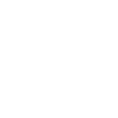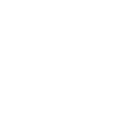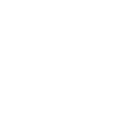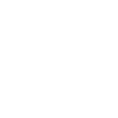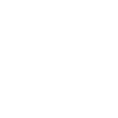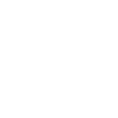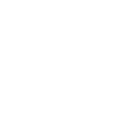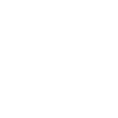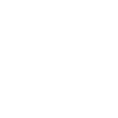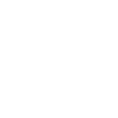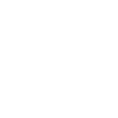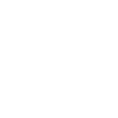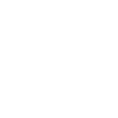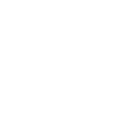Good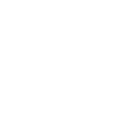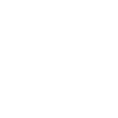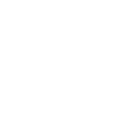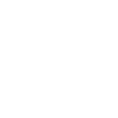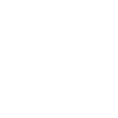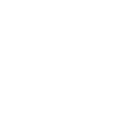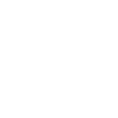Very Good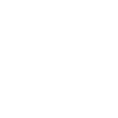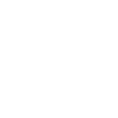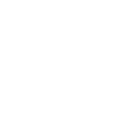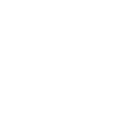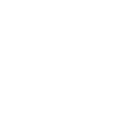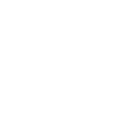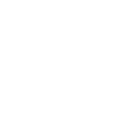Fair